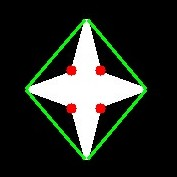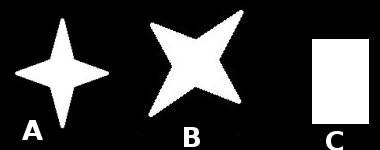# OpenCV-Python 系列 二十三 | 轮廓：更多属性

– 凸性缺陷以及如何找到它们
– 查找点到多边形的最短距离
– 匹配不同的形状

### 理论和代码

#### 1. 凸性缺陷

OpenCV有一个函数来找到这个,cv.convexityDefects()。一个基本的函数调用如下:

hull = cv.convexHull(cnt,returnPoints = False)
defects = cv.convexityDefects(cnt,hull)


import cv2 as cv
import numpy as np
img_gray = cv.cvtColor(img,cv.COLOR_BGR2GRAY)
ret,thresh = cv.threshold(img_gray, 127, 255,0)
contours,hierarchy = cv.findContours(thresh,2,1)
cnt = contours
hull = cv.convexHull(cnt,returnPoints = False)
defects = cv.convexityDefects(cnt,hull)
for i in range(defects.shape):
s,e,f,d = defects[i,0]
start = tuple(cnt[s])
end = tuple(cnt[e])
far = tuple(cnt[f])
cv.line(img,start,end,[0,255,0],2)
cv.circle(img,far,5,[0,0,255],-1)
cv.imshow('img',img)
cv.waitKey(0)
cv.destroyAllWindows()#### 2. 点多边形测试

dist = cv.pointPolygonTest(cnt,(50,50),True)


#### 3. 形状匹配

OpenCV附带一个函数cv.matchShapes()，该函数使我们能够比较两个形状或两个轮廓，并返回一个显示相似性的度量。结果越低，匹配越好。它是根据矩值计算出来的。不同的测量方法在文档中有解释。

import cv2 as cv
import numpy as np
ret, thresh = cv.threshold(img1, 127, 255,0)
ret, thresh2 = cv.threshold(img2, 127, 255,0)
contours,hierarchy = cv.findContours(thresh,2,1)
cnt1 = contours
contours,hierarchy = cv.findContours(thresh2,2,1)
cnt2 = contours
ret = cv.matchShapes(cnt1,cnt2,1,0.0)
print( ret )– 匹配的图像A与本身= 0.0
– 匹配图像A与图像B = 0.001946
– 匹配图像A与图像C = 0.326911

Hu矩是平移、旋转和比例不变的七个矩。第七个是无偏斜量。这些值可以使用cpu.HuMoments()函数找到。

### 练习

1. 检查文档中的cv.pointPolygonTest()，您可以找到红色和蓝色的漂亮图像。它表示从所有像素到白色曲线的距离。曲线内的所有像素都是蓝色的，这取决于距离。外面的点也是红色的。轮廓边缘用白色标记。所以问题很简单。编写一个代码来创建这样的距离表示。

2. 使用cv.matchShapes()比较数字或字母的图像。(这是迈向OCR的简单一步)